# Penrose tiling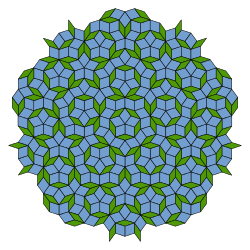A Penrose tiling

A Penrose tiling is an example of non-periodic tiling generated by an aperiodic set of prototiles. Penrose tilings are named after mathematician and physicist Roger Penrose, who investigated these sets in the 1970s. The aperiodicity of prototiles implies that a shifted copy of a tiling will never match the original. A Penrose tiling may be constructed so as to exhibit both reflection symmetry and fivefold rotational symmetry, as in the diagram at the right.

A Penrose tiling has many remarkable properties, most notably:

• It is non-periodic, which means that it lacks any translational symmetry.
• It is self-similar, so the same patterns occur at larger and larger scales. Thus, the tiling can be obtained through "inflation" (or "deflation") and every finite patch from the tiling occurs infinitely many times.
• It is a quasicrystal: implemented as a physical structure a Penrose tiling will produce Bragg diffraction and its diffractogram reveals both the fivefold symmetry and the underlying long range order.

Various methods to construct Penrose tilings have been discovered, including matching rules, substitutions or subdivision rules, cut and project schemes and coverings.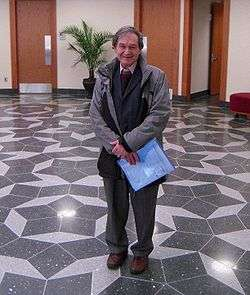Roger Penrose in the foyer of the Mitchell Institute for Fundamental Physics and Astronomy, Texas A&M University, standing on a floor with a Penrose tiling

## Background and history

### Periodic and aperiodic tilings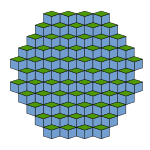Figure 1. Part of a periodic tiling

Penrose tilings are simple examples of aperiodic tilings of the plane. A tiling is a covering of the plane by tiles with no overlaps or gaps; the tiles normally have a finite number of shapes, called prototiles, and a set of prototiles is said to admit a tiling or tile the plane if there is a tiling of the plane using only tiles congruent to these prototiles. The most familiar tilings (e.g., by squares or triangles) are periodic: a perfect copy of the tiling can be obtained by translating all of the tiles by a fixed distance in a given direction. Such a translation is called a period of the tiling; more informally, this means that a finite region of the tiling repeats itself in periodic intervals. If a tiling has no periods it is said to be non-periodic. A set of prototiles is said to be aperiodic if it tiles the plane but every such tiling is non-periodic; tilings by aperiodic sets of prototiles are called aperiodic tilings.

### Earliest aperiodic tilings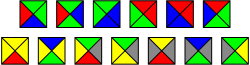The subject of aperiodic tilings received new interest in the 1960s when logician Hao Wang noted connections between decision problems and tilings. In particular, he introduced tilings by square plates with colored edges, now known as Wang dominoes or tiles, and posed the "Domino Problem": to determine whether a given set of Wang dominoes could tile the plane with matching colors on adjacent domino edges. He observed that if this problem were undecidable, then there would have to exist an aperiodic set of Wang dominoes. At the time, this seemed implausible, so Wang conjectured no such set could exist.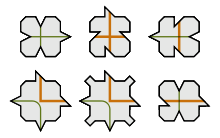Robinson's six prototiles

Wang's student Robert Berger proved that the Domino Problem was undecidable (so Wang's conjecture was incorrect) in his 1964 thesis, and obtained an aperiodic set of 20426 Wang dominoes. He also described a reduction to 104 such prototiles; the latter did not appear in his published monograph, but in 1968, Donald Knuth detailed a modification of Berger's set requiring only 92 dominoes.

The color matching required in a tiling by Wang dominoes can easily be achieved by modifying the edges of the tiles like jigsaw puzzle pieces so that they can fit together only as prescribed by the edge colorings. Raphael Robinson, in a 1971 paper which simplified Berger's techniques and undecidability proof, used this technique to obtain an aperiodic set of just six prototiles.

### Development of the Penrose tilings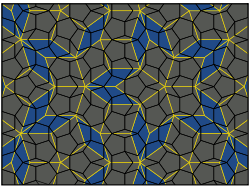Figure 2. The pentagonal Penrose tiling (P1) drawn in black on a colored rhombus tiling (P3) with yellow edges.

The first Penrose tiling (tiling P1 below) is an aperiodic set of six prototiles, introduced by Roger Penrose in a 1974 paper, but it is based on pentagons rather than squares. Any attempt to tile the plane with regular pentagons necessarily leaves gaps, but Johannes Kepler showed, in his 1619 work Harmonices Mundi, that these gaps can be filled using pentagrams (star polygons), decagons and related shapes. Traces of these ideas can also be found in the work of Albrecht Dürer. Acknowledging inspiration from Kepler, Penrose found matching rules (which can be imposed by decorations of the edges) for these shapes, obtaining an aperiodic set. His tiling can be viewed as a completion of Kepler's finite Aa pattern.

Penrose subsequently reduced the number of prototiles to two, discovering the kite and dart tiling (tiling P2 below) and the rhombus tiling (tiling P3 below). The rhombus tiling was independently discovered by Robert Ammann in 1976. Penrose and John H. Conway investigated the properties of Penrose tilings, and discovered that a substitution property explained their hierarchical nature; their findings were publicized by Martin Gardner in his January 1977 "Mathematical Games column" in Scientific American.

In 1981, De Bruijn explained a method to construct Penrose tilings from five families of parallel lines as well as a "cut and project method", in which Penrose tilings are obtained as two-dimensional projections from a five-dimensional cubic structure. In this approach, the Penrose tiling is viewed as a set of points, its vertices, while the tiles are geometrical shapes obtained by connecting vertices with edges.

## The Penrose tilings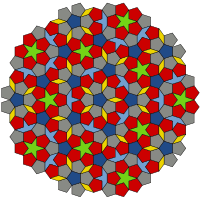A P1 tiling using Penrose's original set of six prototiles

The three types of Penrose tiling, P1P3, are described individually below. They have many common features: in each case, the tiles are constructed from shapes related to the pentagon (and hence to the golden ratio), but the basic tile shapes need to be supplemented by matching rules in order to tile aperiodically; these rules may be described using labeled vertices or edges, or patterns on the tile faces alternatively the edge profile can be modified (e.g. by indentations and protrusions) to obtain an aperiodic set of prototiles.

### The original pentagonal Penrose tiling (P1)

Penrose's first tiling uses pentagons and three other shapes: a five-pointed "star" (a pentagram), a "boat" (roughly 3/5 of a star) and a "diamond" (a thin rhombus). To ensure that all tilings are non-periodic, there are matching rules that specify how tiles may meet each other, and there are three different types of matching rule for the pentagonal tiles. It is common to indicate the three different types of pentagonal tiles using three different colors, as in the figure above right.

### Kite and dart tiling (P2)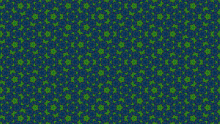Wallpaper created by applying several deflations to a Penrose tiling of type P2 (kite and dart).

Penrose's second tiling uses quadrilaterals called the "kite" and "dart", which may be combined to make a rhombus. However, the matching rules prohibit such a combination. Both the kite and dart are composed of two triangles, called Robinson triangles, after 1975 notes by Robinson.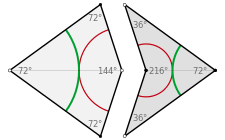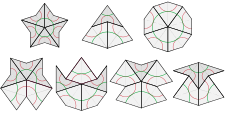Kite and dart tiles (top) and the seven possible vertex figures in a P2 tiling.
• The kite is a quadrilateral whose four interior angles are 72, 72, 72, and 144 degrees. The kite may be bisected along its axis of symmetry to form a pair of acute Robinson triangles (with angles of 36, 72 and 72 degrees).
• The dart is a non-convex quadrilateral whose four interior angles are 36, 72, 36, and 216 degrees. The dart may be bisected along its axis of symmetry to form a pair of obtuse Robinson triangles (with angles of 36, 36 and 108 degrees), which are smaller than the acute triangles.

The matching rules can be described in several ways. One approach is to color the vertices (with two colors, e.g., black and white) and require that adjacent tiles have matching vertices. Another is to use a pattern of circular arcs (as shown above left in green and red) to constrain the placement of tiles: when two tiles share an edge in a tiling, the patterns must match at these edges.

These rules often force the placement of certain tiles: for example, the concave vertex of any dart is necessarily filled by two kites. The corresponding figure (center of the top row in the lower image on the left) is called an "ace" by Conway; although it looks like an enlarged kite, it does not tile in the same way. Similarly the concave vertex formed when two kites meet along a short edge is necessarily filled by two darts (bottom right). In fact, there are only seven possible ways for the tiles to meet at a vertex; two of these figures namely, the "star" (top left) and the "sun" (top right) have 5-fold dihedral symmetry (by rotations and reflections), while the remainder have a single axis of reflection (vertical in the image). All of these vertex figures, apart from the ace and the sun, force the placement of additional tiles.

### Rhombus tiling (P3)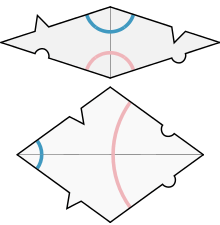Matching rule for Penrose rhombs using circular arcs or edge modifications

The third tiling uses a pair of rhombuses (often referred to as "rhombs" in this context) with equal sides but different angles. Ordinary rhombus-shaped tiles can be used to tile the plane periodically, so restrictions must be made on how tiles can be assembled: no two tiles may form a parallelogram, as this would allow a periodic tiling, but this constraint is not sufficient to force aperiodicity, as figure 1 above shows.

There are two kinds of tile, both of which can be decomposed into Robinson triangles.

• The thin rhomb t has four corners with angles of 36, 144, 36, and 144 degrees. The t rhomb may be bisected along its short diagonal to form a pair of acute Robinson triangles.
• The thick rhomb T has angles of 72, 108, 72, and 108 degrees. The T rhomb may be bisected along its long diagonal to form a pair of obtuse Robinson triangles; in contrast to the P2 tiling, these are larger than the acute triangles.

The matching rules distinguish sides of the tiles, and entail that tiles may be juxtaposed in certain particular ways but not in others. Two ways to describe these matching rules are shown in the image on the right. In one form, tiles must be assembled such that the curves on the faces match in color and position across an edge. In the other, tiles must be assembled such that the bumps on their edges fit together.

There are 54 cyclically ordered combinations of such angles that add up to 360 degrees at a vertex, but the rules of the tiling allow only seven of these combinations to appear (although one of these arises in two ways).

The various combinations of angles and facial curvature allow construction of arbitrarily complex tiles, such as the Penrose chickens.

## Features and constructions

### The golden ratio and local pentagonal symmetry

Several properties and common features of the Penrose tilings involve the golden ratio φ = (1+√5)/2 (approximately 1.618). This is the ratio of chord lengths to side lengths in a regular pentagon, and satisfies φ = 1 + 1/φ.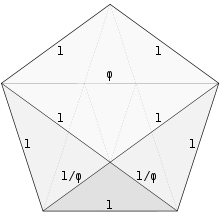Pentagon with an inscribed thick rhomb (light), acute Robinson triangles (lightly shaded) and a small obtuse Robinson triangle (darker). Dotted lines give additional edges for inscribed kites and darts.

Consequently, the ratio of the lengths of long sides to short sides in the (isosceles) Robinson triangles is φ:1. It follows that the ratio of long side lengths to short in both kite and dart tiles is also φ:1, as are the length ratios of sides to the short diagonal in the thin rhomb t, and of long diagonal to sides in the thick rhomb T. In both the P2 and P3 tilings, the ratio of the area of the larger Robinson triangle to the smaller one is φ:1, hence so are the ratios of the areas of the kite to the dart, and of the thick rhomb to the thin rhomb. (Both larger and smaller obtuse Robinson triangles can be found in the pentagon on the left: the larger triangles at the top the halves of the thick rhomb have linear dimensions scaled up by φ compared to the small shaded triangle at the base, and so the ratio of areas is φ2:1.)

Any Penrose tiling has local pentagonal symmetry, in the sense that there are points in the tiling surrounded by a symmetric configuration of tiles: such configurations have fivefold rotational symmetry about the center point, as well as five mirror lines of reflection symmetry passing through the point, a dihedral symmetry group. This symmetry will generally preserve only a patch of tiles around the center point, but the patch can be very large: Conway and Penrose proved that whenever the colored curves on the P2 or P3 tilings close in a loop, the region within the loop has pentagonal symmetry, and furthermore, in any tiling, there are at most two such curves of each color that do not close up.

There can be at most one center point of global fivefold symmetry: if there were more than one, then rotating each about the other would yield two closer centers of fivefold symmetry, which leads to a mathematical contradiction. There are only two Penrose tilings (of each type) with global pentagonal symmetry: for the P2 tiling by kites and darts, the center point is either a "sun" or "star" vertex.

### Inflation and deflation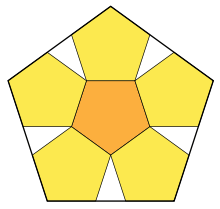A pentagon decomposed into six smaller pentagons (half a dodecahedral net) with gaps

Many of the common features of Penrose tilings follow from a hierarchical pentagonal structure given by substitution rules: this is often referred to as inflation and deflation, or composition and decomposition, of tilings or (collections of) tiles. The substitution rules decompose each tile into smaller tiles of the same shape as those used in the tiling (and thus allow larger tiles to be "composed" from smaller ones). This shows that the Penrose tiling has a scaling self-similarity, and so can be thought of as a fractal.

Penrose originally discovered the P1 tiling in this way, by decomposing a pentagon into six smaller pentagons (one half of a net of a dodecahedron) and five half-diamonds; he then observed that when he repeated this process the gaps between pentagons could all be filled by stars, diamonds, boats and other pentagons. By iterating this process indefinitely he obtained one of the two P1 tilings with pentagonal symmetry.

#### Robinson triangle decompositions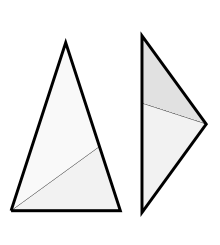Robinson triangles and their decompositions

The substitution method for both P2 and P3 tilings can be described using Robinson triangles of different sizes. The Robinson triangles arising in P2 tilings (by bisecting kites and darts) are called A-tiles, while those arising in the P3 tilings (by bisecting rhombs) are called B-tiles. The smaller A-tile, denoted AS, is an obtuse Robinson triangle, while the larger A-tile, AL, is acute; in contrast, a smaller B-tile, denoted BS, is an acute Robinson triangle, while the larger B-tile, BL, is obtuse.

Concretely, if AS has side lengths (1, 1, φ), then AL has side lengths (φ, φ, 1). B-tiles can be related to such A-tiles in two ways:

• If BS has the same size as AL then BL is an enlarged version φAS of AS, with side lengths (φ, φ, φ2 = 1 + φ) this decomposes into an AL tile and AS tile joined along a common side of length 1.
• If instead BL is identified with AS, then BS is a reduced version (1/φ)AL of AL with side lengths (1/φ,1/φ,1) joining a BS tile and a BL tile along a common side of length 1 then yields (a decomposition of) an AL tile.

In these decompositions, there appears to be an ambiguity: Robinson triangles may be decomposed in two ways, which are mirror images of each other in the (isosceles) axis of symmetry of the triangle. In a Penrose tiling, this choice is fixed by the matching rules furthermore, the matching rules also determine how the smaller triangles in the tiling compose to give larger ones.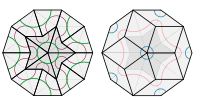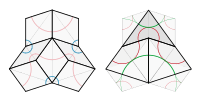Partial inflation of star to yield rhombs, and of a collection of rhombs to yield an ace.

It follows that the P2 and P3 tilings are mutually locally derivable: a tiling by one set of tiles can be used to generate a tiling by another for example a tiling by kites and darts may be subdivided into A-tiles, and these can be composed in a canonical way to form B-tiles and hence rhombs. The P2 and P3 tilings are also both mutually locally derivable with the P1 tiling (see figure 2 above).

The decomposition of B-tiles into A-tiles may be written

BS = AL, BL = AL + AS

(assuming the larger size convention for the B-tiles), which can be summarized in a substitution matrix equation:Combining this with the decomposition of enlarged φA-tiles into B-tiles yields the substitutionso that the enlarged tile φAL decomposes into two AL tiles and one AS tiles. The matching rules force a particular substitution: the two AL tiles in a φAL tile must form a kite thus a kite decomposes into two kites and a two half-darts, and a dart decomposes into a kite and two half-darts. Enlarged φB-tiles decompose into B-tiles in a similar way (via φA-tiles).

Composition and decomposition can be iterated, so that, for exampleThe number of kites and darts in the nth iteration of the construction is determined by the nth power of the substitution matrix:where Fn is the nth Fibonacci number. The ratio of numbers of kites to darts in any sufficiently large P2 Penrose tiling pattern therefore approximates to the golden ratio φ. A similar result holds for the ratio of the number of thick rhombs to thin rhombs in the P3 Penrose tiling.

#### Deflation for P2 and P3 tilings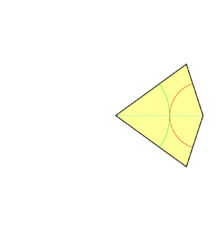Consecutive deflations of the 'sun' vertex in a Penrose tiling of type P2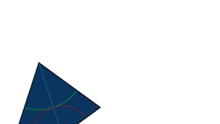Consecutive deflations of a tile-set in a Penrose tiling of type P2 filling a 16:9 wide rectangle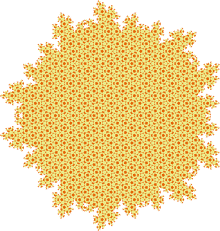8th deflation of the 'sun' vertex in a Penrose tiling of type P2

Starting with a collection of tiles from a given tiling (which might be a single tile, a tiling of the plane, or any other collection), deflation proceeds with a sequence of steps called generations. In one generation of deflation, each tile is replaced with two or more new tiles that are scaled-down versions of tiles used in the original tiling. The substitution rules guarantee that the new tiles will be arranged in accordance with the matching rules. Repeated generations of deflation produce a tiling of the original axiom shape with smaller and smaller tiles.

This rule for dividing the tiles is a subdivision rule.

Name Initial tiles Generation 1 Generation 2 Generation 3
Half-kite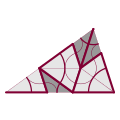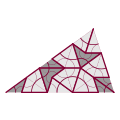Half-dart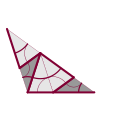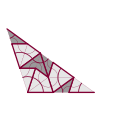Sun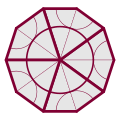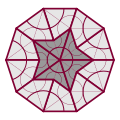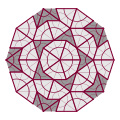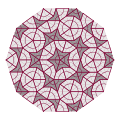Star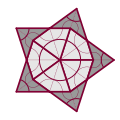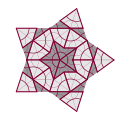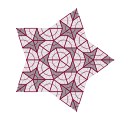#### Consequences and applications

Inflation and deflation yield a method for constructing kite and dart (P2) tilings, or rhombus (P3) tilings, known as up-down generation.

The Penrose tilings, being non-periodic, have no translational symmetry the pattern cannot be shifted to match itself over the entire plane. However, any bounded region, no matter how large, will be repeated an infinite number of times within the tiling. Therefore, a finite patch cannot differentiate between the uncountably many Penrose tilings, nor even determine which position within the tiling is being shown.

This shows in particular that the number of distinct Penrose tilings (of any type) is uncountably infinite. Up-down generation yields one method to parameterize the tilings, but other methods use Ammann bars, pentagrids, or cut and project schemes.

### Decagonal coverings and quasicrystals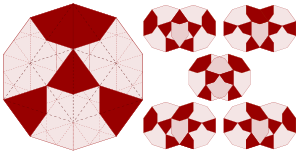Gummelt's decagon (left) with the decomposition into kites and darts indicated by dashed lines; the thicker darker lines bound an inscribed ace and thick rhomb; possible overlaps (right) are by one or two red aces.

In 1996, German mathematician Petra Gummelt demonstrated that a covering (so called to distinguish it from a non-overlapping tiling) equivalent to the Penrose tiling can be constructed using a single decagonal tile if two kinds of overlapping regions are allowed. The decagonal tile is decorated with colored patches, and the covering rule allows only those overlaps compatible with the coloring. A suitable decomposition of the decagonal tile into kites and darts transforms such a covering into a Penrose (P2) tiling. Similarly, a P3 tiling can be obtained by inscribing a thick rhomb into each decagon; the remaining space is filled by thin rhombs.

These coverings have been considered as a realistic model for the growth of quasicrystals: the overlapping decagons are 'quasi-unit cells' analogous to the unit cells from which crystals are constructed, and the matching rules maximize the density of certain atomic clusters.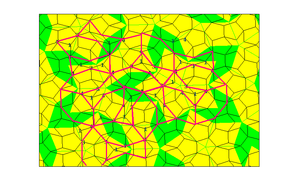Tie and Navette tiling (in red on a Penrose background)

The three variants of the Penrose tiling are mutually locally derivable. Selecting some subsets from the vertices of a P1 tiling allows to produce other non-periodic tilings. If the corners of one pentagon in P1 are labeled in succession by 1,3,5,2,4 an unambiguous tagging in all the pentagons is established, the order being either clockwise or counterclockwise. Points with the same label define a tiling by Robinson triangles while points with the numbers 3 and 4 on them define the vertices of a Tie-and-Navette tiling.

There are also other related unequivalent tilings, such as the hexagon-boat-star and MikullaRoth tilings. For instance, if the matching rules for the rhombus tiling are reduced to a specific restriction on the angles permitted at each vertex, a binary tiling is obtained. Its underlying symmetry is also fivefold but it is not a quasicrystal. It can be obtained either by decorating the rhombs of the original tiling with smaller ones, or by applying substitution rules, but not by de Bruijn's cut-and-project method.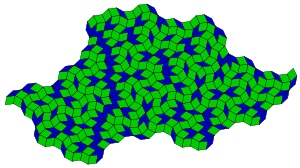A variant tiling which is not a quasicrystal. It is not a Penrose tiling because it does not comply with the tile alignment rules.

### Penrose tilings and art

The aesthetic value of tilings has long been appreciated, and remains a source of interest in them; here the visual appearance (rather than the formal defining properties) of Penrose tilings has attracted attention. The similarity with some decorative patterns used in the Middle East has been noted; the physicists Peter J. Lu and Paul Steinhardt have presented evidence that a Penrose tiling underlies some examples of medieval Islamic geometric patterns, such as tilings at the Darb-e Imam shrine in Isfahan.

Drop City artist Clark Richert used Penrose rhombs in artwork in 1970 - derived by projecting the rhombic triacontahedron shadow onto a plane observing the embedded "fat" rhombi and "skinny" rhombi which tile together to produce the non-periodic tessellation.. Art historian Martin Kemp has observed that Albrecht Dürer sketched similar motifs of a rhombus tiling.

San Francisco's new \$4.2 billion Transbay Transit Center is planned to have perforations in its exterior's undulating white metal skin in the Penrose pattern.

The floor of the atrium of the Molecular and Chemical Sciences Building at the University of Western Australia is tiled with Penrose tiles.

The Andrew Wiles Building, the location of the Mathematics Department at the University of Oxford as of October 2013, includes a section of Penrose tiling as the paving of its entrance. The pedestrian part of the street Keskuskatu in Helsinki is paved using a form of Penrose tiling. The work was finished in 2014.

1. General references for this article include Gardner 1997, pp. 130, Grünbaum & Shephard 1987, pp. 520548 & 558579, and Senechal 1996, pp. 170206.
2. Gardner 1997, pp. 20, 23
3. Grünbaum & Shephard 1987, p. 520
4. Culik & Kari 1997
5. Wang 1961
6. Austin 2005a
7. Berger 1966
8. Grünbaum & Shephard 1987, p. 584
9. Gardner 1997, p. 5
10. Robinson 1971
11. Grünbaum & Shephard 1987, p. 525
12. Senechal 1996, pp. 173–174
13. Penrose 1974
14. Grünbaum & Shephard 1987, section 2.5
15. Luck 2000
16. Senechal 1996, p. 171
17. Gardner 1997, p. 6
18. Gardner 1997, p. 19
19. Gardner 1997, chapter 1
20. de Bruijn 1981
21. The P1P3 notation is taken from Grünbaum & Shephard 1987, section 10.3
22. Grünbaum & Shephard 1987, section 10.3
23. Penrose 1978, p. 32
24. "However, as will be explained momentarily, differently colored pentagons will be considered to be different types of tiles." Austin 2005a; Grünbaum & Shephard 1987, figure 10.3.1, shows the edge modifications needed to yield an aperiodic set of prototiles.
25. "The rhombus of course tiles periodically, but we are not allowed to join the pieces in this manner." Gardner 1997, pp. 67
26. Grünbaum & Shephard 1987, pp. 537 547
27. Senechal 1996, p. 173
28. Gardner 1997, p. 8
29. Gardner 1997, pp. 1011
30. Gardner 1997, p. 12
31. Senechal 1996, p. 178
32. Gardner 1997, p. 9
33. Gardner 1997, p. 27
34. Grünbaum & Shephard 1987, p. 543
35. In Grünbaum & Shephard 1987, the term "inflation" is used where other authors would use "deflation" (followed by rescaling). The terms "composition" and "decomposition", which many authors also use, are less ambiguous.
36. Ramachandrarao P., On the fractal nature of Penrose tiling, Current Science 79(2000) p364
37. Grünbaum & Shephard 1987, p. 546
38. Senechal 1996, pp. 157158
39. Austin 2005b
40. Senechal 1996, p. 183
41. Gardner 1997, p. 7
42. "... any finite patch that we choose in a tiling will lie inside a single inflated tile if we continue moving far enough up in the inflation hierarchy. This means that anywhere that tile occurs at that level in the hierarchy, our original patch must also occur in the original tiling. Therefore, the patch will occur infinitely often in the original tiling and, in fact, in every other tiling as well." Austin 2005a
43. Lord & Ranganathan 2001
44. Gummelt 1996
45. Steinhardt & Jeong 1996; see also Steinhardt, Paul J. "A New Paradigm for the Structure of Quasicrystals".
46. Luck R., "Penrose Sublattices", Journal of Non Crystalline Solids 117-8 (90)832-5
47. Lançon & Billard 1988
48. Godrèche & Lançon 1992; see also E. Harriss and D. Frettlöh. "Binary". Tilings Encyclopedia. Department of Mathematics, University of Bielefeld.
49. Prange, Sebastian R.; Peter J. Lu (2009-09-01). "The Tiles of Infinity". Saudi Aramco World. Aramco Services Company. pp. 24–31. Retrieved 2010-02-22.
50. Lu & Steinhardt 2007
51. Kemp 2005
52. Kuchar, Sally (July 11, 2013), "Check Out the Proposed Skin for the Transbay Transit Center", Curbed
53. http://www.treasures.uwa.edu.au/treasures/66/
54. http://www.hs.fi/tiede/a1407209514060

### Secondary sourcesWikimedia Commons has media related to Penrose tilings.